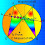## Saturday, February 20, 2016

### Geometry Problem 1189: Circle, Tangent Line, Secant, Chord, Collinear Points

Geometry Problem. Post your solution in the comment box below.
Level: Mathematics Education, High School, Honors Geometry, College.

Click the figure below to view more details of problem 1189.1.http://s10.postimg.org/i7q916o6x/pro_1189.png

See below for basic properties of complete quadrilateral and pole and polar of a circle .
1. ADEGFP is a complete quadrilateral with diagonals DG meet EF at H and PH meet DE and FG at N and M ( see sketch) then (ANDE)=(AMFG)=-1 or AD/AE=ND/NE and AF/AG=MF/MG .
2. A secant from A meet circle O at D and E and cut BC (polar of A) at N then (ANDE)=-1 or AD/AE= ND/NE.
Conversely if exist a point N on DE such that (ANDE)=-1 then N will be on BC.

Applying these properties to our problem, PH cut DE at N and FG at M then (ANDE)=(AMFG)= -1 (property #1) and M and N will be on BC (polar of pole A) (property #2) . so B, C and H are collinear.

1.Unfortunately Peter Tran's link does not work, so I don't know the identity of point P which is not on your drawing.
Thanks for helping.

2.See below for the link of problem 1189

https://photos.app.goo.gl/r2trcF4VxiqcWSjs8

Peter Tran

2.Polar point A is BC,Η is conjugate to A.AND A located in BC.

3.Dear Antonio - do u have another proof for this?

Perhaps extension of Pascal's Theorem?

1.2.Some hints?

4.Пусть P, пересечение описанная окружность FOE и описанная окружность DOG, <DEG=a, и <FGE=b.
<EPG=<EPO+<GPO.
По свойства окружность, <EPO=<EFO=90-b, и <GPO=<GDO=90-a, значит
<EPG=180-a-b=<EAG, значит точки E,P,A,G лежит на одной окружность.
Таким образом <APG=<AEG=a потому что EPAG вписанный четырехугольник.
<OPA=<GPO+<APG=(90-a)+(a)=90 по предыдущий выводы.
Значит что P также лежит на окружность пересекающий точки B, A, C, O.
Относительно окружность O, EF инверсии окружность FOE, DG инверсии окружность DOG, BC инверсии окружность BACO, и H инверсии P. Если P лежит на окружность BACO, H должен лежит на BC.

5.is Mr. Bazarov's solution correct?

1.can somebody translate the solution to English ?

2.Dear Peter, following Ivan's solution using "Google Translate":

Let P, the intersection of the circumscribed circle and the circumscribed circle FOE DOG, <DEG = a, and <FGE = b.
<EPG = <EPO + <GPO.
According to the properties of a circle, <EPO = <EFO = 90-b, and <GPO = <GDO = 90-a, then <EPG = 180-a-b = <EAG, then point E, P, A, G lies on a circle.
Thus <APG = <AEG = a because EPAG inscribed quadrilateral.
<OPA = <GPO + <APG = (90-a) + (a) = 90 on the previous findings.
This means that P also lies on a circle intersecting points B, A, C, O.
Regarding the circumference O, EF inversion circumference FOE, DG inversion DOG circumference, BC BACO circumference inversion and inversion H P.
If P lies on the circle BACO, should H It lies on BC.

6.Let's denote the intersection of EG and DF as X. Clearly BC is the polar of A. Also from Brokard's theorem we have that HX is the polar of A. So B, C, H and X are all collinear since they lay on the polar of A.

7.I would like to see a proof with elementary euclidean geometry without using poles and polars.

Thank you very much.

Manlio